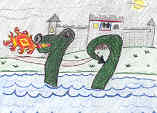# 79

This number is a prime.

Just showing those entries submitted by 'Gaydos': (Click here to show all)Kari Phillips ©2003The sum of the first 79 brilliant numbers is divisible by 79 (44003/79=557 which is also a prime). [Gaydos]79 permutations of the 6 non-prime digits (014689) are prime numbers, counting 0 as a permissible first digit. [Gaydos]Country singer Merle Haggard died on his 79th birthday. [Gaydos]There are 79 strobogrammatic numbers with sums of reciprocals of digits that are less than one. [Gaydos]The 79th triangular number (3160) is the first to have all four triangular digits. [Gaydos]The 79th triangular number (3160) has a Collatz trajectory length of 79. [Gaydos]

(There are 6 curios for this number that have not yet been approved by an editor.)